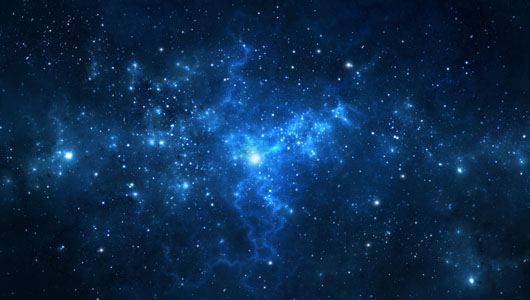# Cosmic Energy

In Dark Energy by Brian Koberlein4 Comments

A while back I wrote about the relationship between the distance of a galaxy from us and the speed at which it moves away from us.  This relationship is now known as Hubble’s law, after Edwin Hubble, who first plotted the speed of a couple dozen galaxies versus their distance in 1929.  What he found was a linear relationship between speed and distance.  In other words, it seemed the speed of a galaxy divided by its distance was a constant, now known as the Hubble constant.  It was the first solid evidence of an expanding universe.

Since Hubble’s day we’ve been able to measure the speeds and distances of more than four thousand galaxies, so we’re able to get a much better measurement of the Hubble constant.  All this extra data has made things very interesting.

For one thing, as we measure more distant galaxies we have to change Hubble’s law a bit.  Hubble’s original relation was between speed and distance, but at really large distances galaxies are moving away from us at a large fraction of the speed of light.  This means we have to take special relativity into account at large distances.  For this reason the Hubble relation is now expressed not in terms of galactic speed, but in terms of a measure of redshift known as z.  The nice thing about z is that it allows special relativity to be basically factored out of observations.  If Hubble’s model holds, then a plot of z versus distance should be a straight line.

A wonderful aspect of observational astronomy is that when you look at more and more distant objects, you are also looking further back into time.  If a galaxy is a billion light years away from us, the light we observe left that galaxy a billion years ago.  This means galactic distance is a also a measure of the past.  As a result, the Hubble constant is not just a measure z versus distance, but also a measure of z versus time.  In other words it tells us the speed at which the universe is expanding.

In the past decade, however, we’ve found that Hubble’s constant doesn’t quite hold, as you can see in the figure above.  The linear relation is plotted as a black line, but the best fit to the data is the red line.  The red line isn’t straight, but instead curves slightly upward. This means at really large distances the redshift is greater than we would expect.  The expansion speed of the universe is not constant, but is getting larger.  In other words, the universe is accelerating.

Of course this means something is likely causing this acceleration. That something is known as dark energy.

1.Hi a very informative series of articles thanks very much, one question though in the plot of z against distance, surely as distance increase, you are measuring something that increasingly happened in the past ie the distance axis has a hidden time element and so if a galaxy had slowed down since the light we are looking at left it, we wouldn’t know yet. Acceleration is clearly time dependent and so if you are not comparing speeds at the same point in time you can’t say that the universe is accelerating decelerating or at a constant speed? Sorry I am an engineer not a physicist.

1.Author

Actually, the redshift is just one point of evidence for cosmic expansion. We can also do things such as measure the brightness of a galaxy vs. distance, or its apparent size. All of these results are compared to models, and the model that best fits is the cosmic expansion model.

2.I see in picture you assume redshift as doppler shift but there is no evidence that it is doppler shift and it could be non-doppler redshift.

1.Author

The image is from a book I wrote, where the Doppler shift is being discussed, hence the vertical axis label. For galactic redshift, however, most of it is not due to any relative motion, but rather due to cosmic expansion.